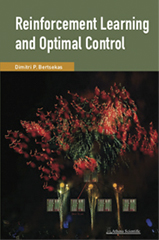# Reinforcement Learning and Optimal Control

ISBN: 978-1-886529-39-7
Publication: 2019, 388 pages, hardcover
Price: \$89.00
AVAILABLEThis book considers large and challenging multistage decision problems, which can be solved in principle by dynamic programming (DP), but their exact solution is computationally intractable. We discuss solution methods that rely on approximations to produce suboptimal policies with adequate performance. These methods are collectively known by several essentially equivalent names: reinforcement learning, approximate dynamic programming, and neuro-dynamic programming. They have been at the forefront of research for the last 25 years, and they underlie, among others, the recent impressive successes of self-learning in the context of games such as chess and Go.

Our subject has benefited greatly from the interplay of ideas from optimal control and from artificial intelligence, as it relates to reinforcement learning and simulation-based neural network methods. One of the aims of the book is to explore the common boundary between these two fields and to form a bridge that is accessible by workers with background in either field. Another aim is to organize coherently the broad mosaic of methods that have proved successful in practice while having a solid theoretical and/or logical foundation. This may help researchers and practitioners to find their way through the maze of competing ideas that constitute the current state of the art.

This book relates to several of our other books: Neuro-Dynamic Programming (Athena Scientific, 1996), Dynamic Programming and Optimal Control (4th edition, Athena Scientific, 2017), Abstract Dynamic Programming (2nd edition, Athena Scientific, 2018), and Nonlinear Programming (3rd edition, Athena Scientific, 2016).

However, the mathematical style of this book is somewhat different. While we provide a rigorous, albeit short, mathematical account of the theory of finite and infinite horizon dynamic programming, and some fundamental approximation methods, we rely more on intuitive explanations and less on proof-based insights. Moreover, our mathematical requirements are quite modest: calculus, a minimal use of matrix-vector algebra, and elementary probability (mathematically complicated arguments involving laws of large numbers and stochastic convergence are bypassed in favor of intuitive explanations).

The book illustrates the methodology with many examples and illustrations, and uses a gradual expository approach, which proceeds along four directions:

• From exact DP to approximate DP: We first discuss exact DP algorithms, explain why they may be difficult to implement, and then use them as the basis for approximations.

• From finite horizon to infinite horizon problems: We first discuss finite horizon exact and approximate DP methodologies, which are intuitive and mathematically simple, and then progress to infinite horizon problems.

• From deterministic to stochastic models: We often discuss separately deterministic and stochastic problems, since deterministic problems are simpler and offer special advantages for some of our methods.

• From model-based to model-free implementations: We first discuss model-based implementations, and then we identify schemes that can be appropriately modified to work with a simulator.

The author is McAfee Professor of Engineering at the Massachusetts Institute of Technology and a member of the prestigious US National Academy of Engineering. He is the recipient of the 2001 A. R. Raggazini ACC education award, the 2009 INFORMS expository writing award, the 2014 Kachiyan Prize, the 2014 AACC Bellman Heritage Award, the 2015 SIAM/MOS George B. Dantsig Prize. In 2018, he shared the John von Neumann INFORMS theory award with John Tsitsiklis for the books "Neuro-Dynamic Programming", and "Parallel and Distributed Computation".